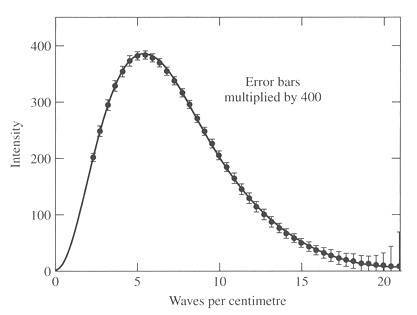# Cosmic microwave background radiation

## Homework Statement

The cosmic microwave background radiation has a blackbody type spectrum. Determine its max frequency and the correspondent wavelenght. Verify if found frequency is a microwave frequency and compare with the following curve:## Homework Equations

Using the Wien Law displacemente, and knowing that CMBR has a thermal black body spectrum at a temperature of 2.725 K. We can easily calculate the wavelength peak and its frequency.

λmax = 1,06mm

frequency = λmax/c = 2,83E11HZ

We can verify that the frequency that we obtained corresponds to the microwave frequency.

But when i am comparing with this curve, i did not find any relation...

I just do not know what is the relation of the graphic with the obtained results.

Anybody to help? Help is always appreciated.!!!

Last edited:

## Answers and Replies

mfb
Mentor
That is a tricky problem.

The curve shows the frequency-dependence of the intensity (waves/cm is proportional to frequency) - you can calculate the wavelength which corresponds to its peak, and it will not agree with your wavelength.

This is not an error on your side, it is a surprising mathematical result: If you switch between wavelength and frequency dependence, you get different positions of the peaks, as "per wavelength" on the y-axis is different from "per frequency".

That is a tricky problem.

The curve shows the frequency-dependence of the intensity (waves/cm is proportional to frequency) - you can calculate the wavelength which corresponds to its peak, and it will not agree with your wavelength.

This is not an error on your side, it is a surprising mathematical result: If you switch between wavelength and frequency dependence, you get different positions of the peaks, as "per wavelength" on the y-axis is different from "per frequency".

So, the difference is the switching from wavelength to frequency dependence. The results are "mathematically" equal?

mfb
Mentor
The intensity maximum of the wavelength is different from intensity maximum of the frequency - even if the curves correspond to the same spectrum.

Understood!

Thanks a lot for the help!